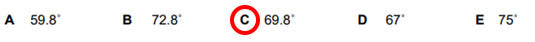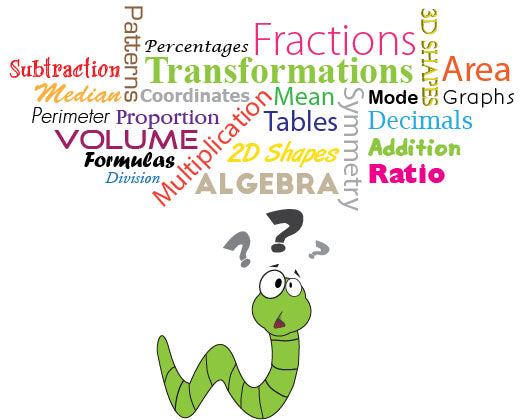We are currently dispatching orders as normal, but delivery times may be affected Find a rep
Free tools and resources for primary and secondary to support mental and physical wellbeing

# How can I best support my child for mathematics in the 11+ exams?Chris Pearse is a qualified Primary School Teacher with 10 years' experience in teaching. He started Teachitright in 2006 to provide support for children taking secondary school exams and is passionate about helping children achieve their potential whilst enjoying education.

There are a wide range of topics covered in an 11+ maths tests. This article will attempt to summarise these mathematical areas, while also providing a set of comprehensive tips to developing your child’s overall mathematical ability.

Everyone has a different perception on maths and this often stems from our maths lessons at school. Some kids find it interesting and inspiring, while others it strikes dread into their hearts! Sometimes, pupils find it hard to access the 11+ maths curriculum, especially if they don’t have the foundations. A prime example of this prior knowledge is knowing your times tables off by heart. Interestingly, the rote learning often experienced by previous generations is making a comeback and those pupils who can recall number facts automatically seem to love maths.

Maths is often about establishing relationships between numbers and relating numeracy to real-life situations can make this subject more relevant to children. An example might be teaching ratios using smarties and having the chance to eat the sweets at the end of the session! There are so many opportunities in everyday life to bring maths alive.Cooking with children can support an understanding of weight, measurement, counting or time. Eating pizza can be the perfect opportunity to teach fractions in a fun way!

If students can see the huge potential maths skills can provide this might help them dismiss that mathematics is boring. If a child sees that maths is involved in so many careers. Examples, include video game designer, fashion designer or engineer. As mentioned at the beginning of this blog your own negativity towards the subject must not be filtered into your own children. Understanding maths is everywhere from getting ready for school, shopping for groceries or even working out how many points your favourite sports team needs for promotion, can show children how much maths matters.

One of the main skills required for an 11+ maths section, is the ability to answer word problems. These can often be multi-step and require students to choose the correct operations to calculate the answers. See an example below:Firstly, it is important to read the whole question and consider the steps required. In the question above it is worth working backwards. What temperature do we need to convert to Fahrenheit? Therefore, add 50% of 14 to establish the Celsius temperature, 21°C. Next, multiplying 1.8 by 21 = 37.8. Finally, adding 37.8 to 32 = 69.8Many of the 11+ tests are multiple-choice and encouraging your child to estimate before solving the question can either help eliminate possible answer options and provide a valuable clue to the approximation of the outcome. If your answer is a long way from your estimation, it might imply you’ve made an error. If this happens, like a detective, retrace your tracks and double check each calculation. Taking the time to practise these types of questions is vital as most questions are presented in this format rather than purely calculations. Wrapping the numbers with words can cause children to panic in an exam situation, so reading through examples together can really help them break it down into manageable steps. In some exam boards pupils use previous answers to inform their next question and this further emphasises the need to get the first question correct, if questions/numbers are connected.

Delving deeper into the eleven plus maths topics reveals that children can be tested on algebra questions. Unsurprisingly, this is not simultaneous equations, but children do need to have a strong grasp of the basic algebraic rules. This topic might not be taught until year 6 or beyond, hence the need to cover this area with your child. Below an example of a typical question:

Sasha is working with formula 5n³ - 3. What is n when the output she gets is 317?

﻿These are the jottings which accompany the above question:
(layout as an equation to be solved)
5n³ - 3 = 317 (+3 to both sides of the equation)
5n³ = 320 (divide both sides by 5)
n³ = 64
n x n x n = 64
n = 4

In the contrary to the above topic area, most concepts are covered in the maths national curriculum. The only main difference is the questions are slightly more advanced. One of the major areas covered in an 11+ test is data handling/statistics. This can involve children interpreting graphs, charts or tables. Familiarity of these ways to present information are really important. Again, relating it to real-life situations, for instance looking at a plane timetable together with your child and being able to decipher the data. How long between the New York and Bologna flight? Record your answer in minutes.Showing children how to interpret a Venn diagram or Carroll diagram can be useful. Generally, pupils at school are interested in science and this can be a great chance to teach maths, without them being aware they are applying their numeracy skills. This can be demonstrated by investigating the topic of friction using stopping distances of toy cars on different slanted surfaces (plastic, cotton, wood or aluminium). Measuring the distances in millimetres, centimetres and metres, converting between the different units. This leads into another imperative element of the 11+ maths sections which is equivalence. Pupils need to be confident with equivalent values and this can be demonstrated below with a comparison question.Being able to convert between percentages, decimals and fractions can help compare values much easier if they are all under the same units. In the above question we can convert them into any of the mentioned but usually it saves time to adopt the most dominant values. In this example they are converted to decimals:

0.66    0.68    0.66̇    0.09    0.6

Therefore, we can establish 0.68 Is the largest value.

Unfortunately, it is not possible in a short article to cover every aspect of mathematics in the 11+. However, outlined below is a comprehensive list and the skills involved.

Working with numbers
You’ll need to use addition, subtraction, multiplication or division to answer these questions (or all four to solve some mixed calculations). You’ll need to use place value and rounding to solve number problems.

Number Knowledge
You’ll need to be able to work with fractions, percentages and decimals and you’ll need to understand ratio, proportion and a bit about different types of numbers.Number problems
You’ll need to look for number patterns as well as use formulas and algebraic expressions. You could be given word problems where you need to use information in the question to find the answer.

Data Handling
Some questions use data in tables and graphs. You’ll need to be able to find information in a table and know how to read different types of graphs. You also need to be able to find the mode, median, mean, range of a data set and to work with probabilities.

Shape and Space
You may be tested on the names and properties of 2d and 3d shapes. Other questions may ask about symmetry, coordinates, transformations, volume, area and perimeter. You might also have to imagine shapes being rotated to solve shape problems.

You may be tested on units and time. You’ll need to be able to read scales and convert between different units (e.g. mm and cm)

Mixed problems
There might also be questions that cover more than one topic. For example, you may be given a question where you have to read a graph and do some calculations.

In summary, mathematics should be taught in context and using practical activities where possible to help children understand how maths can be applied in the real world. Developing a confidence with working with number is also vital. Remembering the key to performing well in an 11+ maths paper or section is having the core numeracy skills. These areas include basic arithmetic, times tables and efficient methods in order to access the more advanced maths problems. Using those everyday opportunities to explore maths with children can really bring the subject to life!

Find out more about Teachitright: https://www.teachitright.com/